# Vignette for phyloseq: Analysis of high-throughput microbiome census data

If you find phyloseq and/or its tutorials useful, please acknowledge and cite phyloseq in your publications:

phyloseq: An R package for reproducible interactive analysis and graphics of microbiome census data (2013) PLoS ONE 8(4):e61217 http://dx.plos.org/10.1371/journal.pone.0061217

## Other resources

The phyloseq project also has a number of supporting online resources, most of which can by found at the phyloseq home page, or from the phyloseq stable release page on Bioconductor.

To post feature requests or ask for help, try the phyloseq Issue Tracker.

## Summary

The analysis of microbiological communities brings many challenges: the integration of many different types of data with methods from ecology, genetics, phylogenetics, network analysis, visualization and testing. The data itself may originate from widely different sources, such as the microbiomes of humans, soils, surface and ocean waters, wastewater treatment plants, industrial facilities, and so on; and as a result, these varied sample types may have very different forms and scales of related data that is extremely dependent upon the experiment and its question(s). The phyloseq package is a tool to import, store, analyze, and graphically display complex phylogenetic sequencing data that has already been clustered into Operational Taxonomic Units (OTUs), especially when there is associated sample data, phylogenetic tree, and/or taxonomic assignment of the OTUs. This package leverages many of the tools available in R for ecology and phylogenetic analysis (vegan, ade4, ape, picante), while also using advanced/flexible graphic systems (ggplot2) to easily produce publication-quality graphics of complex phylogenetic data. phyloseq uses a specialized system of S4 classes to store all related phylogenetic sequencing data as single experiment-level object, making it easier to share data and reproduce analyses. In general, phyloseq seeks to facilitate the use of R for efficient interactive and reproducible analysis of OTU-clustered high-throughput phylogenetic sequencing data.

A separate vignette is included within the phyloseq-package that describes the basics of importing pre-clustered phylogenetic sequencing data, data filtering, as well as some transformations and some additional details about the package and installation. A quick way to load it is:

vignette("phyloseq-basics")


By contrast, this vignette is intended to provide functional examples of the analysis tools and wrappers included in phyloseq. All necessary code for performing the analysis and producing graphics will be included with its description, and the focus will be on the use of example data that is included and documented within the phyloseq-package.

library("phyloseq")
library("ggplot2")


And because we will show examples of custom modifications to ggplot2 plots, we also loaded ggplot2 as well. Here are my favorite ggplot2 theming commands. These are completely optional, and modifiable.

theme_set(theme_bw())
pal = "Set1"
scale_colour_discrete <- function(palname = pal, ...) {
scale_colour_brewer(palette = palname, ...)
}
scale_fill_discrete <- function(palname = pal, ...) {
scale_fill_brewer(palette = palname, ...)
}


## Data

### Interface with the microbio.me/qiime server

See the microbio_me_qiime tutorial for more details and examples downloading and importing into phyloseq/R directly from this public database.

### Included Data

To facilitate testing and exploration of tools in phyloseq, this package includes example data from published studies. Many of the examples in this vignette use either the Global Patterns or enterotype datasets as source data. The Global Patterns data was described in a 2011 article in PNAS(Caporaso 2011), and compares the microbial communities of 25 environmental samples and three known “mock communities” — a total of 9 sample types — at a depth averaging 3.1 million reads per sample. The human enterotype dataset was described in a 2011 article in Nature (Arumugam 2011), which compares the faecal microbial communities from 22 subjects using complete shotgun DNA sequencing. The authors further compare these microbial communities with the faecal communities of subjects from other studies, for a total of 280 faecal samples / subjects, and 553 genera. Sourcing data from different studies invariable leads to gaps in the data for certain variables, and this is easily handled by R's coreNA features.

Because this data is included in the package, the examples can easily be run on your own computer using the code shown in this vignette. The data is loaded into memory using the data command. Let's start by loading the Global Patterns data.

data(GlobalPatterns)


Later on we will use an additional categorical designation — human versus non-human associated samples — that was not in the original dataset. Now is a good time to add it as an explicit variable of the sample_data, and because we don't want to type long words over and over, we'll choose a shorter name for this modified version of Global Patterns, call it GP, and also remove a handful of taxa that are not present in any of the samples included in this dataset (probably an artifact):

# prune OTUs that are not present in at least one sample
GP <- prune_taxa(taxa_sums(GlobalPatterns) > 0, GlobalPatterns)
# Define a human-associated versus non-human categorical variable:
human <- get_variable(GP, "SampleType") %in% c("Feces", "Mock", "Skin", "Tongue")
# Add new human variable to sample data:
sample_data(GP)$human <- factor(human)  ## Simple exploratory graphics ### Easy Richness Estimates For further details, see the plot_richness tutorial We can easily create a complex graphic that compares the richness estimates of samples from different environment types in the Global Patterns dataset, using the plot_richness function. Note that it is important to use raw (untrimmed) OTU-clustered data when performing richness estimates, as they can be highly dependent on the number of singletons in a sample. alpha_meas = c("Observed", "Chao1", "ACE", "Shannon", "Simpson", "InvSimpson") (p <- plot_richness(GP, "human", "SampleType", measures=alpha_meas))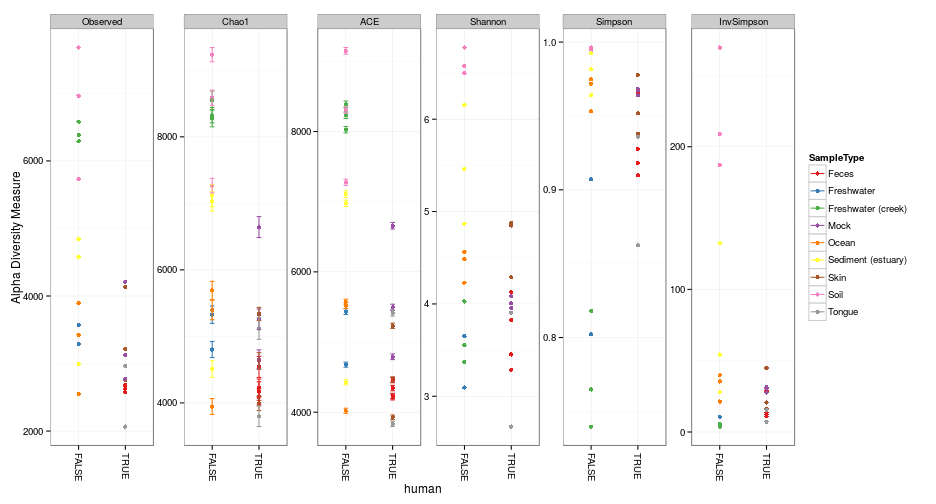Add a ggplot2 box plot layer to the previous plot p + geom_boxplot(data=p$data, aes(x=human, y=value, color=NULL), alpha=0.1)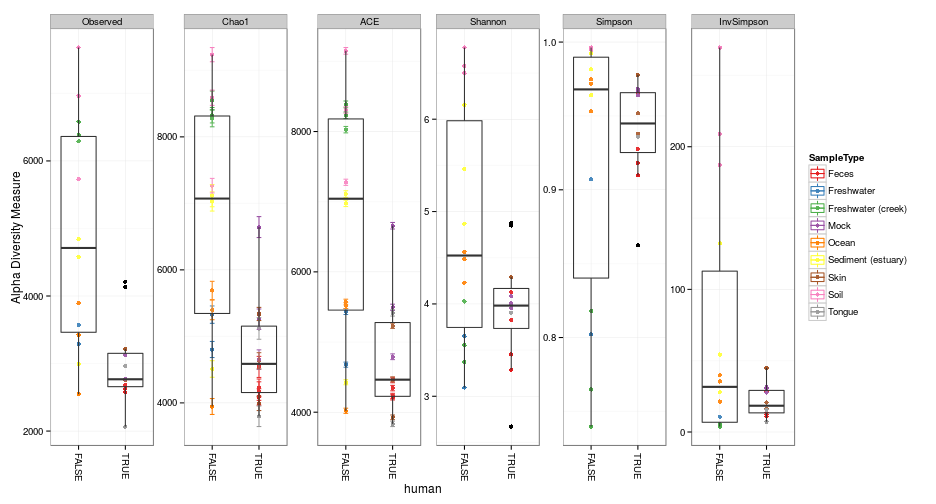### Exploratory tree plots

For further details, see the plot_tree tutorial

phyloseq also contains a method for easily plotting an annotated phylogenetic tree with information regarding the sample in which a particular taxa was observed, and optionally the number of individuals that were observed.

For the sake of creating a readable tree, let's subset the data to just the Chlamydiae phylum, which consists of obligate intracellular pathogens and is present in only a subset of environments in this dataset.

GP.chl <- subset_taxa(GP, Phylum=="Chlamydiae")


And now we will create the tree graphic form this subset of Global Patterns, shading by the “SampleType” variable, which indicates the environment category from which the microbiome samples originated. The following command also takes the option of labeling the number of individuals observed in each sample (if at all) of each taxa. The symbols are slightly enlarged as the number of individuals increases.

plot_tree(GP.chl, color="SampleType", shape="Family", label.tips="Genus", size="Abundance")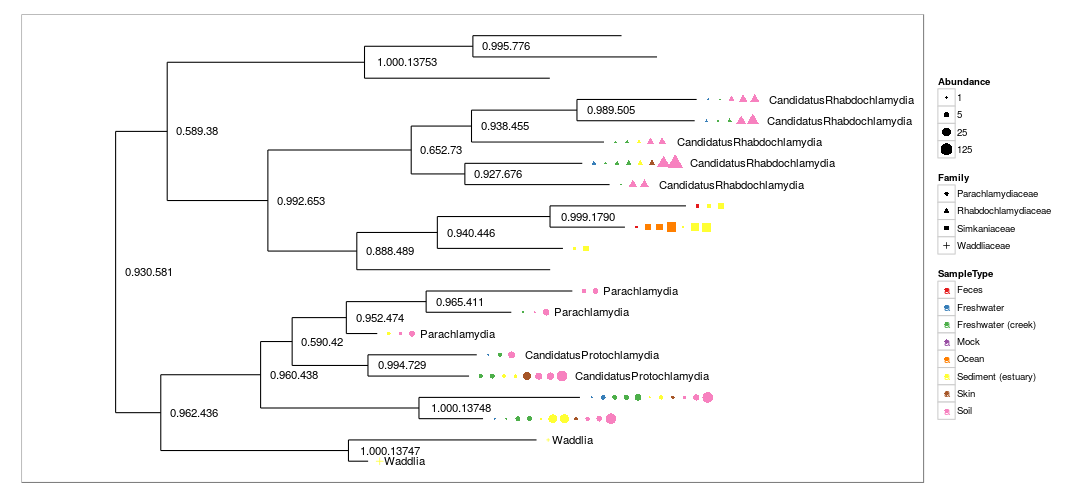Phylogenetic tree representation of the Chlamydiae species in the microbiome samples of the “Global Patterns” dataset(Caporaso 2011).

### Exploratory bar plots

For further details, see the plot_bar tutorial

In the following example we use the included “enterotype” dataset (Arumugam 2011).

data(enterotype)


We start with a simple rank-abundance barplot, using the cumulative fractional abundance of each OTU in the dataset. In the enterotype dataset, the available published data are simplified as sample-wise fractional occurrences, rather than counts of individuals\footnote{Unfortunate, as this means we lose information about the total number of reads and associated confidences, ability to do more sophisticated richness estimates, etc. For example, knowing that we observed 1 sequence read of a species out of 100 total reads means something very different from observing 10,000 reads out of 1,000,000 total., and OTUs are clustered/labeled at the genus level, but no other taxonomic assignment is available. In this barplot we further normalized by the total number of samples (280).

par(mar = c(10, 4, 4, 2) + 0.1) # make more room on bottom margin
N <- 30
barplot(sort(taxa_sums(enterotype), TRUE)[1:N]/nsamples(enterotype), las=2)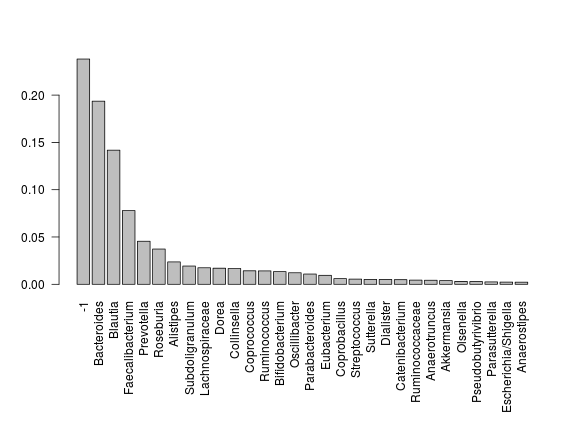###### An example exploratory barplot using base R graphics and thetaxa_sums and nsamples functions.

Note that this first barplot is clipped at the 30th OTU. This was chosen because ntaxa(enterotype) =553 OTUs would not be legible on the plot. As you can see, the relative abundances have decreased dramatically by the 10th-ranked OTU.

So what are these OTUs? In the enterotype dataset, only a single taxonomic rank type is present:

rank_names(enterotype)

##  "Genus"


This means the OTUs in this dataset have been grouped at the level of genera, and no other taxonomic grouping/transformation is possible without additional information (like might be present in a phylogenetic tree, or with further taxonomic classification analysis).

We need to know which taxonomic rank classifiers, if any, we have available to specify in the second barplot function in this example, plot_bar(). We have already observed how quickly the abundance decreases with rank, so wo we will subset the enterotype dataset to the most abundantN taxa in order to make the barplot legible on this page.

TopNOTUs <- names(sort(taxa_sums(enterotype), TRUE)[1:10])
ent10   <- prune_taxa(TopNOTUs, enterotype)
print(ent10)

## phyloseq-class experiment-level object
## otu_table()   OTU Table:         [ 10 taxa and 280 samples ]
## sample_data() Sample Data:       [ 280 samples by 9 sample variables ]
## tax_table()   Taxonomy Table:    [ 10 taxa by 1 taxonomic ranks ]


Note also that there are 280 samples in this dataset, and so a remaining challenge is to consolidate these samples into meaningful groups. A good place to look is the available sample variables, which in most cases will carry more “meaning” than the sample names alone.

sample_variables(ent10)

##  "Enterotype"     "Sample_ID"      "SeqTech"        "SampleID"
##  "Project"        "Nationality"    "Gender"         "Age"
##  "ClinicalStatus"


The parameters to plot_bar in the following code-chunk were chosen after various trials. We suggest that you also try different parameter settings while you're exploring different features of the data. In addition to the variables names of sample_data, the plot_bar() function recognizes the names of taxonomic ranks (if present). See the help documentation and further details in the examples and on the wiki page. In this example we have also elected to organize data by “facets” (separate, adjacent sub-plots) according to the genus of each OTU. Within each genus facet, the data is further separated by sequencing technology, and the enterotype label for the sample from which each OTU originated is indicated by fill color. Abundance values from different samples and OTUs but having the same variables mapped to the horizontal (x) axis are sorted and stacked, with thin horizontal lines designating the boundaries. With this display it is very clear that the choice of sequencing technology had a large effect on which genera were detected, as well as the fraction of OTUs that were assigned to a Genus.

plot_bar(ent10, "SeqTech", fill="Enterotype", facet_grid=~Genus)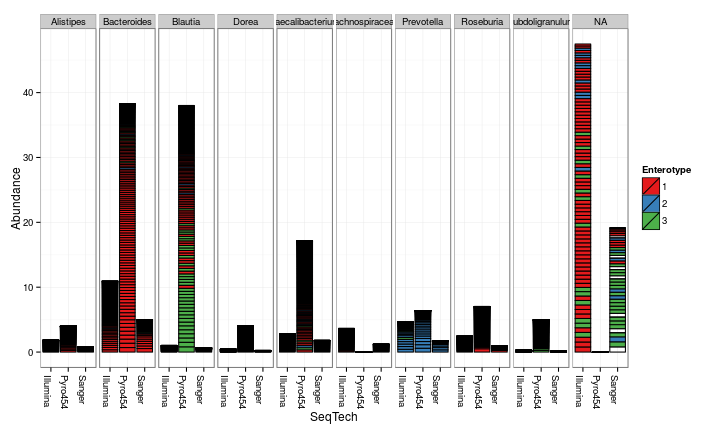An example exploratory bar plot using the plot_bar function. In this case we have faceted the data (abundance values) according to the genera of each OTU. The subset of OTUs that have not been assigned to a specific genus are in the NA panel. Within each facet, the data is further separated by sequencing technology, and each OTU is shaded according to the enterotype of the sample it form which it came. Abundance values from different samples and OTUs but having the same variables mapped to the horizontal (x) axis are sorted and stacked, with thin horizontal lines designating the boundaries.

Figure summarizes quantitatively the increased abundances of Bacteroides and Prevotella in the Enterotypes 1 and 2, respectively. Interestingly, a large relative abundance of Blautia was observed for Enterotype 3, but only from 454-pyrosequencing data sets, not the Illumina or Sanger datasets. This suggests the increased Blautia might actually be an artifact. Similarly, Prevotella appears to be one of the most abundant genera in the Illumina-sequenced samples among Enterotype 3, but this is not reproduced in the 454-pyrosequencing or Sanger sequencing data.

## Exploratory analysis and graphics

### Exploratory Heat Map

For further details, see the plot_heatmap tutorial

As the number of taxa in a dataset gets very large, the ability to effectively display all of the elements of the data becomes compromised, and a heatmap representation is no exception. It can also be time-consuming to render. To address both these issues, we show an example in which we have subsetted the Global Patterns dataset to a manageable portion, in this case, the Crenarchaeota phylum.

data("GlobalPatterns")
gpac <- subset_taxa(GlobalPatterns, Phylum=="Crenarchaeota")
(p <- plot_heatmap(gpac, "NMDS", "bray", "SampleType", "Family"))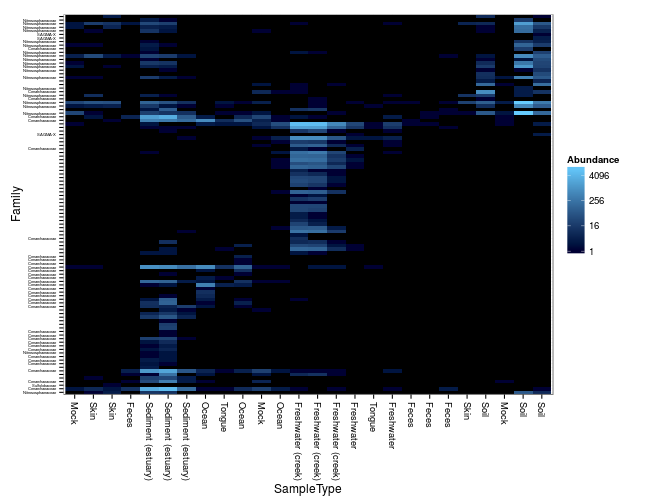What if you wanted to change the axis labels?

p$scales$scales[]$name <- "My X-Axis" p$scales$scales[]$name <- "My Y-Axis"
print(p)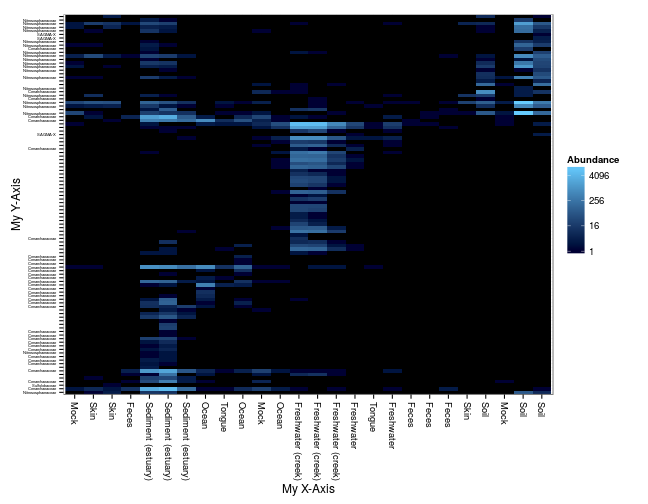Note that it is possible to order the sample/species indices by any of the ordination methods supported in the ordinate function; and also that the color scheme can be modified with additional arguments.

### Microbiome Network Representation

For further details, see the plot_network tutorial

Continuing with the enterotype dataset, here are some examples for creating a custom network representation of the relationship between microbiome samples in an experiment. This relies heavily on the igraph and ggplot2 packages to create a network display of the “connectedness” of samples according to some user-provided ecological similarity. By default, points represent microbiom samples, and are determined using an algorithm that optimizes the clarity of the display of network “edges”, but the spatial position of points does not imply any continuous similarity information like would be the case in an ordination.

In this example, the default dissimilarity index was used (Jaccard, co-occurrence), with a maximum distance of 0.3 required to create an edge. Any function that can operate on phyloseq-objects and return a sample-wise distance can be provided as the dist.fun argument, or a character string of the name of the distance function already supported in phyloseq. Other distances may result in very different clustering, and this is a choice that should be understood and not taken too lightly, although there is little harm in trying many different distances.

Interestingly, at this level of analysis and parameter-settings the two major sub-graphs appear to be best explained by the sequencing technology and not the subject enterotype, suggesting that the choice of sequencing technology has a major effect on the microbial community one can observe. This seems to differ somewhat with the inferences described in the “enterotype” article (Arumugam 2011). However, there could be some confounding or hidden variables that might also explain this phenomenon, and the well-known differences in the sequence totals between the technologies may also be an important factor. Furthermore, since this is clearly an experimental artifact (and they were including data from multiple studies that were not orginally planned for this purpose), it may be that the enterotype observation can also be shown in a network analysis of this data after removing the effect of sequencing technology and related sequencing effort. Such an effort would be interesting to show here, but is not yet included.

data(enterotype)
plot_net(enterotype, maxdist=0.4, color="SeqTech", shape="Enterotype")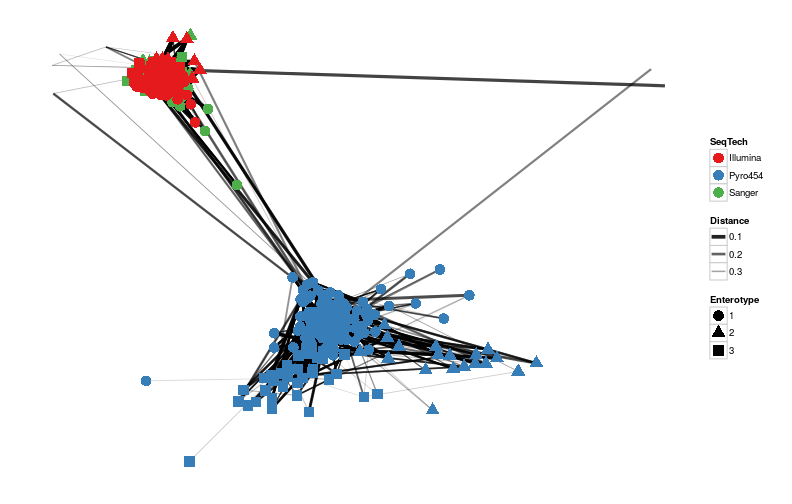Network representation of the relationship between microbiome samples in the “Enterotype” dataset (Arumugam 2011).

### Ordination Methods

For further details, see the plot_ordination tutorial

Ordination methods can be a useful tool for exploring complex phylogenetic sequencing data, particularly when the hypothesized structure of the data is poorly defined (or there isn't a hypothesis). The phyloseq package provides some useful tools for performing ordinations and plotting their results, via the ordinate() andplot_ordination() functions, respectively. Although there are many options and methods supported, a first-step will probably look something like the following:

my.physeq <- import("Biom", BIOMfilename="myBiomFile.biom")
my.ord    <- ordinate(my.physeq)
plot_ordination(my.physeq, my.ord, color="myFavoriteVarible")


It is probably a good idea to read the documentation for these two functions, as they also provide links to related functions and additional examples you can try immediately on your own machine.

help(import)
help(ordinate)
help(distance)
help(plot_ordination)


#### Principal Coordinates Analysis (PCoA)

We take as our first example, a reproduction of Figure 5 from the “Global Patterns” article(Caporaso 2011). The authors show a 3-dimensional representation of the first three axes of a Principal Coordinates Analysis (PCoA; This is also sometimes referred to as “Multi-Dimensional Scaling”, or “MDS”) performed on the unweighted-UniFrac distance using all of the available sequences (their approach included both 5' and 3' sequences). According to the authors, “the first axis [appears to be associated with a] host associated/free living [classification],” and similarly the third axis with “saline/nonsaline environment[s].”

The following reproduces the unweighted UniFrac distance calculation on the full dataset. Note that this calculation can take a long time because of the large number of OTUs. Parallelization is recommended for large datasets, typically if they are as large as Global Patterns, or larger. For details on parallelization, see the details section and examples in the UniFrac() documentation, and also the page dedicated to the topic on the phyloseq-demos site:

http://joey711.github.io/phyloseq-demo/unifrac.html

data(GlobalPatterns)

GPUF <- UniFrac(GlobalPatterns)


Load the pre-computed distance matrix, GPUF

load(system.file("doc", "Unweighted_UniFrac.RData", package="phyloseq"))


Calculate the PCoA on this distance matrix, GPUF.

GloPa.pcoa = ordinate(GlobalPatterns, method="PCoA", distance=GPUF)


Before we look at the results, let's first investigate how much of the total distance structure we will capture in the first few axes. We can do this graphically with a “scree plot”, an ordered barplot of the relative fraction of the total eigenvalues associated with each axis.

plot_scree(GloPa.pcoa, "Scree plot for Global Patterns, UniFrac/PCoA")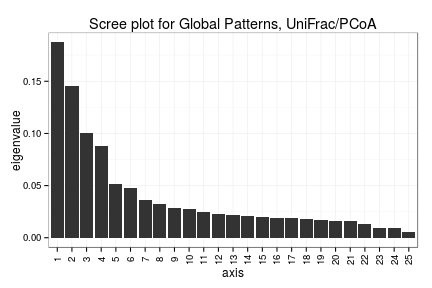Scree plot of the PCoA used to create Figure 5 from the “Global Patterns” article(Caporaso 2011). The first three axes represent 43% of the total variation in the distances. Interestingly, the fourth axis represents another 9%, and so may warrant exploration as well. A scree plot is an important tool for any ordination method, as the relative importance of axes can vary widely from one dataset to another.

Next, we will reproduce Figure 5 from the “Global Patterns” article(Caporaso 2011), but separating the three axes into 2 plots using plot_ordination().

(p12 <- plot_ordination(GlobalPatterns, GloPa.pcoa, "samples", color="SampleType") +
geom_point(size=5) + geom_path() + scale_colour_hue(guide = FALSE) )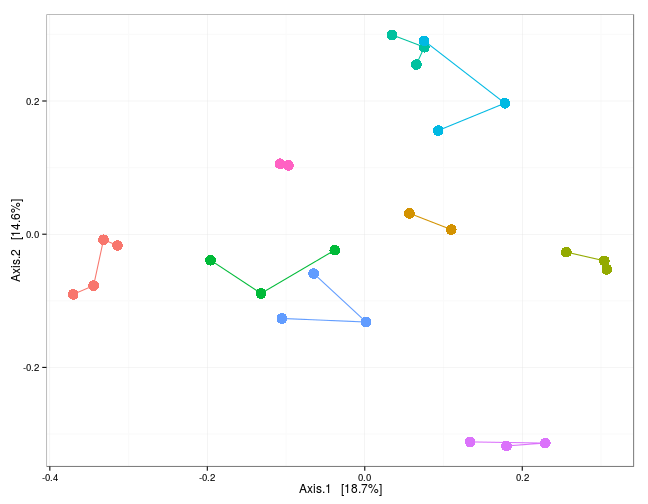(p13 <- plot_ordination(GlobalPatterns, GloPa.pcoa, "samples", axes=c(1, 3),
color="SampleType") + geom_line() + geom_point(size=5) )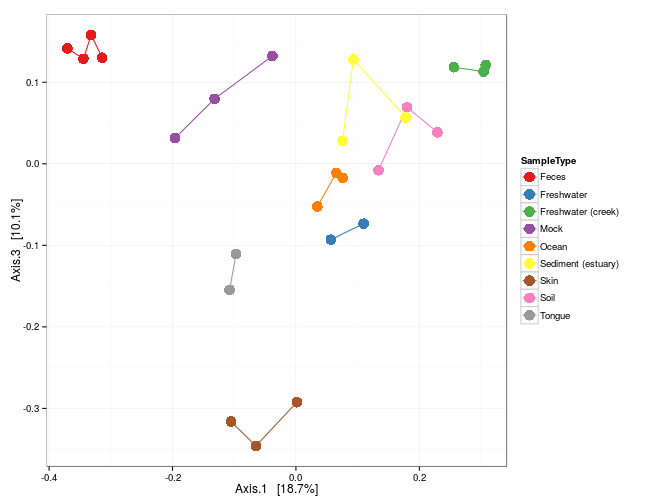#### non-metric Multi-Dimensional Scaling (NMDS)

We repeat the previous example, but instead using non-metric multidimensional scaling (NMDS) limited to just two dimensions. This approach limits the amount of residual distance “not shown” in the first two (or three) axes, but forefeits some mathematical properties and does not always converge within the specified number of axes.

# (Re)load UniFrac distance matrix and GlobalPatterns data
data(GlobalPatterns)
# perform NMDS, set to 2 axes
GP.NMDS <- ordinate(GlobalPatterns, "NMDS", GPUF)

## Run 0 stress 0.1432774
## Run 1 stress 0.167021
## Run 2 stress 0.1432778
## ... procrustes: rmse 0.0004269284  max resid 0.001562517
## *** Solution reached

(p <- plot_ordination(GlobalPatterns, GP.NMDS, "samples", color="SampleType") +
geom_line() + geom_point(size=5) )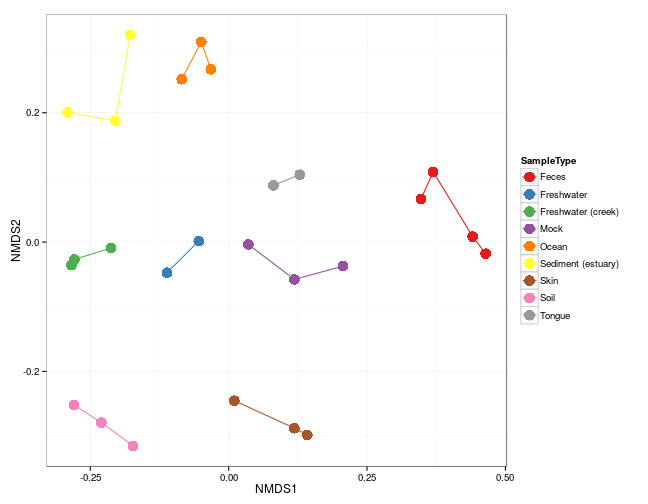###### An example exploratory ordination using non-metric multidimensional scaling (NMDS) on the unweighted UniFrac distance between samples of the “Global Patterns” dataset. Sample points are shaded by environment type, and connected by a line if they belong to the same type. Compare with Figure 5 from the “Global Patterns” article(Caporaso 2011).

The figure nicely shows the relative dissimilarities between microbial communities from different habitats. However, it fails to indicate what was different between the communities. For an ordination method that provides information on the taxa that explain differences between samples (or groups of samples), we use Correspondence Analysis.

#### Correspondence Analysis (CA)

In the following section we will show continue our exploration of the “GlobalPatterns” dataset using various features of an ordination method called Correspondence Analysis. We give special emphasis to exploratory interpretations using the biplot, because it provides additional information that is not available from PCoA or NMDS.

Let's start by performing a Correspondence Analysis and investigating the scree plot. Both interestingly and challengingly, the scree plot suggests that the Global Patterns abundance data is quite high-dimensional, with the first two CA axes accounting for not quite 17% of the total (chi-square) variability. Note the absence of a steep decline in eigenvalue fraction as axis number increases. Each additional axis represents only marginally less variability than the previous. It is often more convenient if the first two (or three) axes account for most of the variability.

First, let's severely subset the number of species for the sake of run-time.\footnote{This is for illustration purposes only, do not repeat unless you are very sure you have a good reason for doing this.

data(GlobalPatterns)
# Take a subset of the GP dataset, top 200 species
topsp <- names(sort(taxa_sums(GlobalPatterns), TRUE)[1:200])
GP    <- prune_taxa(topsp, GlobalPatterns)
# Subset further to top 5 phyla, among the top 200 OTUs.
top5ph <- sort(tapply(taxa_sums(GP), tax_table(GP)[, "Phylum"], sum), decreasing=TRUE)[1:5]
GP     <- subset_taxa(GP, Phylum %in% names(top5ph))
# Re-add human variable to sample data:
sample_data(GP)$human <- factor(human)  Now perform the correspondence analysis. # Now perform a unconstrained correspondence analysis gpca <- ordinate(GP, "CCA") # Scree plot plot_scree(gpca, "Scree Plot for Global Patterns Correspondence Analysis")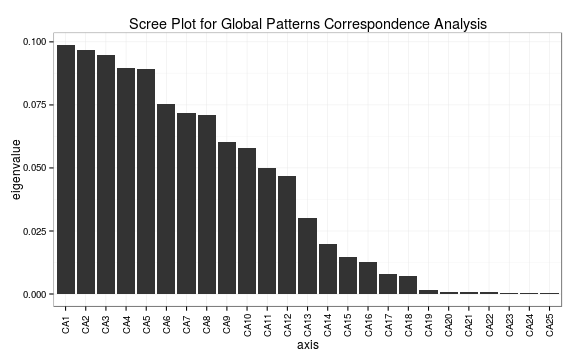The correspondence analysis (CA) scree plot of the “Global Patterns” dataset. Now let's investigate how the samples behave on the first few CA axes. (p12 <- plot_ordination(GP, gpca, "samples", color="SampleType") + geom_line() + geom_point(size=5) )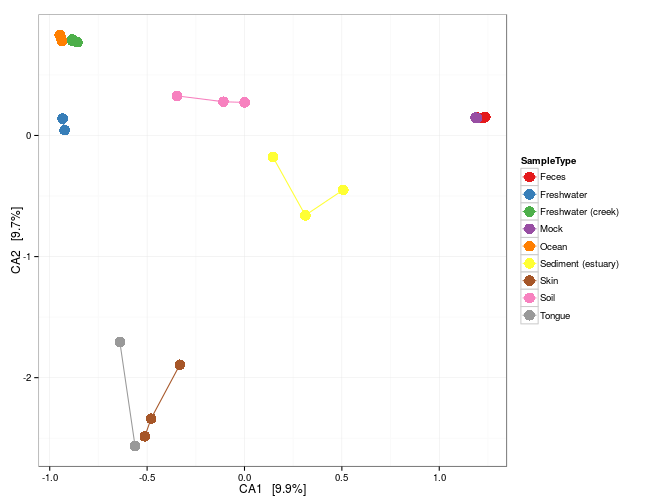(p34 <- plot_ordination(GP, gpca, "samples", axes=c(3, 4), color="SampleType") + geom_line() + geom_point(size=5) )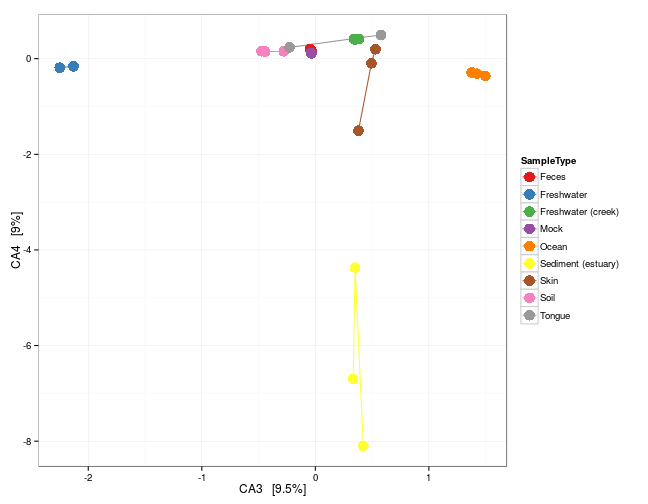First 4 axes of Correspondence Analysis (CA) of the “Global Patterns” dataset (Caporaso 2011). A clear feature of these plots is that the feces and mock communities cluster tightly together, far away from all other samples on the first axis (CA1). The skin and tongue samples separate similarly, but on the second axis. Taken together, it appears that the first two axes are best explained by the separation of human-associated “environments” from the other non-human environments in the dataset, with a secondary separation of tongue and skin samples from feces. We will now investigate further this top-level structure of the data, using an additional feature of correspondence analysis that allows us to compare the relative contributions of individual taxa on the same graphical space: the “biplot”. However, because we just displayed the position of samples in the ordination and there are often many thousands of OTUs, we will focus on creating an interpretable plot of the OTUs. For creating graphics that combine the two plots, try the "biplot" or "split" option for type in plot_ordination(). p1 <- plot_ordination(GP, gpca, "species", color="Phylum") (p1 <- ggplot(p1$data, p1$mapping) + geom_point(size=5, alpha=0.5) + facet_wrap(~Phylum) + scale_colour_hue(guide = FALSE) )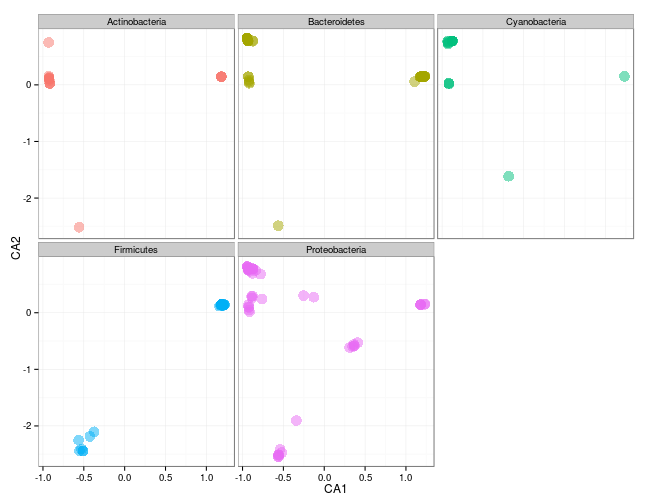Species plot of the “Global Patterns” correspondence analysis first two axes, with each phylum on a different panel (“facet”). Only the most abundant 5 phyla among the most abundant 200 taxa (cumulative, all samples) are included. Arbitrary reduction, for computational efficiency of example. Let's try drawing the figure again, only this time summarizing the species points as a 2D density estimate, without any individual points. (p3 <- ggplot(p1$data, p1$mapping) + geom_density2d() + facet_wrap(~Phylum) + scale_colour_hue(guide = FALSE) )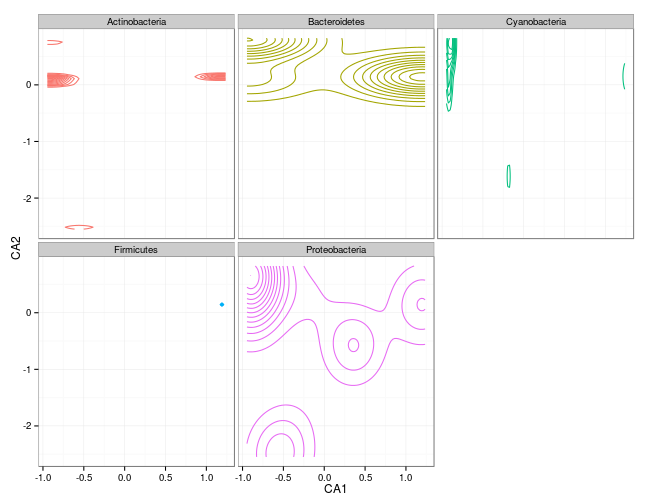Redrawn figure, which is severely overplotted, as a 2-dimensional species-density topographic map, faceted in the same way. These figures reveal some useful patterns and interesting outliers, but what if we want a complete summary of how each phylum is represented along each axis? The following code is a way to show this using boxplots, while still avoiding the occlusion problem (points layered on top of each other), and also conveying some useful information about the pattern of taxa that contribute to the separation of human-associated samples from the other sample types. It re-uses the data that was stored in the ggplot2 plot object created in the previous figure, p, and creates a new boxlot graphical summary of the positions of each phylum. library("reshape2") # Melt the species-data.frame, DF, to facet each CA axis separately mdf <- melt(p1$data[, c("CA1", "CA2", "Phylum", "Family", "Genus")],
id=c("Phylum", "Family", "Genus") )
# Select some special outliers for labelling
LF <- subset(mdf, variable=="CA2" & value < -1.0)
# build plot: boxplot summaries of each CA-axis, with labels
p <- ggplot(mdf, aes(Phylum, value, color=Phylum)) +
geom_boxplot() +
facet_wrap(~variable, 2) +
scale_colour_hue(guide = FALSE) +
theme_bw() +
theme( axis.text.x = element_text(angle = -90, vjust = 0.5) )
# Add the text label layer, and render ggplot graphic
(p <- p + geom_text(data=subset(LF, !is.na(Family)),
mapping = aes(Phylum, value+0.1, color=Phylum, label=Family),
vjust=0,
size=2))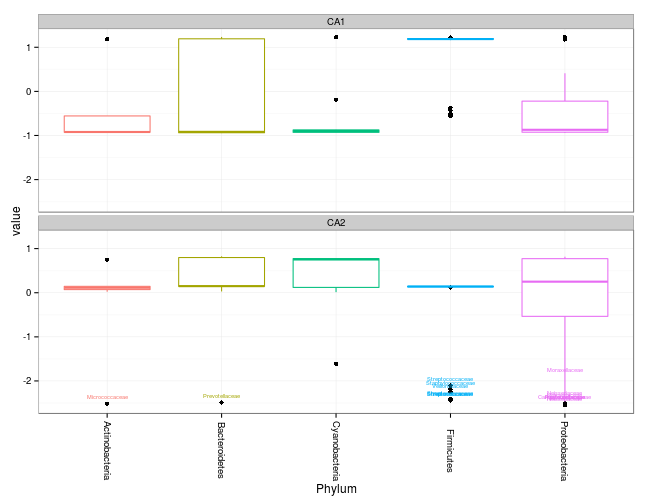###### Boxplot of taxa (species in this case) of the “Global Patterns” CA first two axes, shaded/separated by phylum. Through this approach it is much easier to see particular species that cluster unusually relative to the rest of their phylum, for example the Bacteroidetes species (Prevotellaceae family) that is positioned most in the negative CA2 direction toward the Tongue/Skin samples.

One way to relate some of the high-level patterns we observed from correspondence analysis is to directly visualize the abundances in an organized, quantitative way, to see if this does in fact support / explain the human/environment microbiome differences. Here is an example using the plot_bar function described in an earlier section.

plot_bar(GP, x="human", fill="SampleType", facet_grid= ~ Phylum)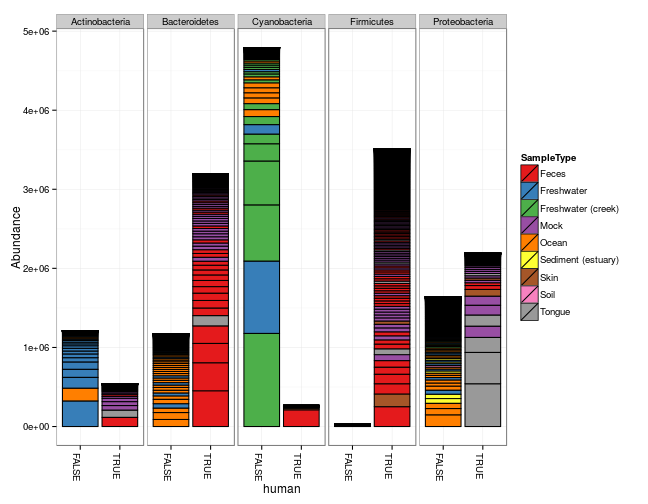###### Phylum-level comparison of relative abundance of taxa in samples that are from human microbiomes (or not).

In this figure we've used the threshold parameter to omit all but phyla accounting for the top 90% of phyla in any one sample. Some patterns emerging from this display appear to be: (1) Cyanobacteria, Actinobacteria appear under-represented in human samples; (2) conversely, Firmicutes appear over-represented in human samples; (3) Acidobacteria, Verrucomicrobia appear over-represented in the fecal samples; (4) the only Crenarchaeota were observed in the Mock sample, which is not really a community but a simulated community used as a control. These are not hugely surprising based on previous biological observations from the field, but it is hopefully useful code that can be applied on other datasets that you might have.

#### Double Principle Coordinate Analysis (DPCoA)

Here is a quick example illustrating the use of Double Principal Coordinate Analysis (DPCoA~\cite{Pavoine2004523), using the using the ordinate() function in phyloseq, as well as the “biplot” option for plot_ordination(). For a description that includes an applied example using the “enterotype” dataset and comparison with UniFrac/PCoA, see Fukuyama et al~\cite{fukuyama2012com.

GP.dpcoa <- ordinate(GP, "DPCoA")
pdpcoa <- plot_ordination(GP, GP.dpcoa, type="biplot",
color="SampleType", shape="Phylum")
shape.fac <- pdpcoa$data[, deparse(pdpcoa$mapping\$shape)]
man.shapes <- c(19, 21:25)
names(man.shapes) <- c("Samples", levels(shape.fac)[levels(shape.fac)!="Samples"])
p2dpcoa <- pdpcoa + scale_shape_manual(values=man.shapes)


A biplot representation of a Double Principal Coordinate Analysis (DPCoA), on a simplified version of the “Global Patterns” dataset with only the most abundant 200 OTUs included.

# Show just Samples or just Taxa
plot_ordination(GP, GP.dpcoa, type="taxa", shape="Phylum")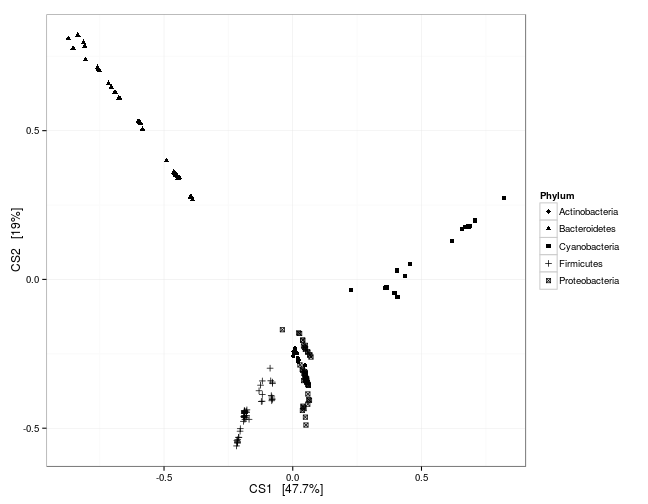plot_ordination(GP, GP.dpcoa, type="samples", color="SampleType")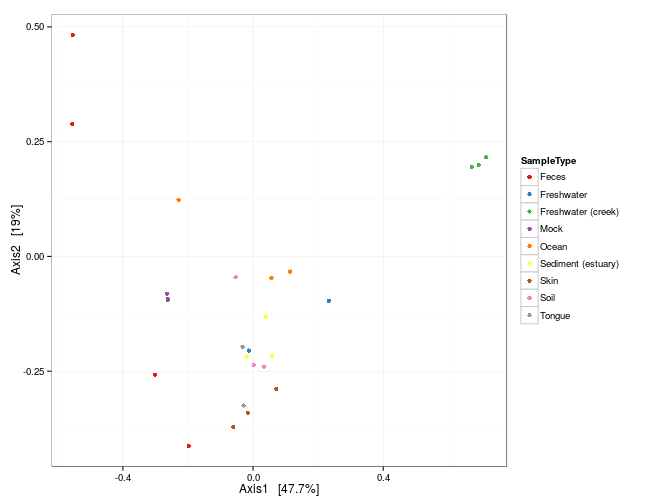# Split
plot_ordination(GP, GP.dpcoa, type="split",
color="SampleType", shape="Phylum") +
ggplot2::scale_colour_discrete()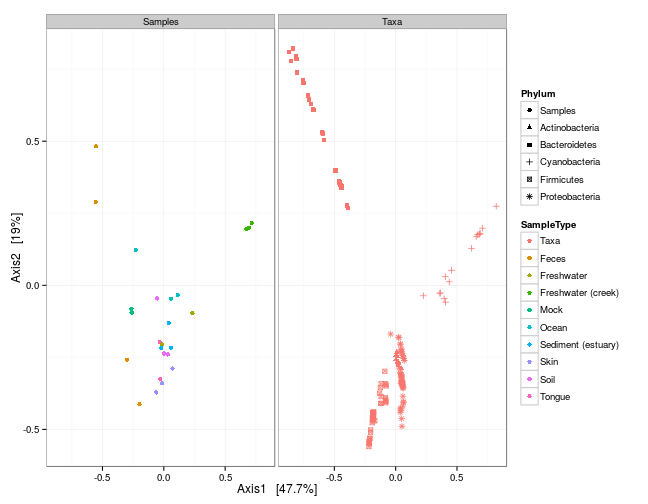### Distance Methods

#### distance(): Central Distance Function

Many comparisons of microbiome samples, including the graphical model and the PCoA analysis, require a calculation for the relative dissimilarity/distance between one microbial community and another. The phyloseq-package provides a general “wrapper” function for calculating ecological distance matrices between the samples in an experiment.

distance() currently supports 43 method options, as well as user-provided arbitrary methods via an interface to vegan's designdist() function. Currrently only sample-wise distances are supported (the type argument), but eventually species-wise (OTU-wise) distances will be supported as well. In addition to supporting any of the method options to the three main distance functions of the vegan-package~\cite{veganpkg — including the 14 distances of the vegdist() function and all 24 ecological distances reviewed in Koleff et al. 2003~\cite{Koleff2003JAE coded by betadiverdistance() will eventually support many of the distance methods in the ade4-package~\cite{Dray:2007vs, and others. It also supports the Fast UniFrac distance function (Section~\ref{sec:unifrac) included in phyloseq as native R code, and a wrapper for retreiving the sample-distances from Double Principal Coordinate Analysis (DPCoA).

The function takes a phyloseq-class object and an argument indicating the distance type; and it returns a dist-class distance matrix.

data(esophagus)
distance(esophagus, "bray")

##           B         C
## C 0.4061135
## D 0.4976303 0.5907173

distance(esophagus, "wunifrac") # weighted UniFrac

##           B         C
## C 0.2035424
## D 0.2603371 0.2477016

distance(esophagus, "jaccard") # vegdist jaccard

##           B         C
## C 0.5776398
## D 0.6645570 0.7427056

distance(esophagus, "g") # betadiver method option "g"

##           B         C
## C 0.6136364
## D 0.6250000 0.6078431


#### UniFrac and weighted UniFrac

UniFrac is a recently-defined~\cite{Lozupone:2005gn and popular distance metric to summarize the difference between pairs of ecological communities. All UniFrac variants use a phylogenetic tree of the relationship among taxa as central information to calculating the distance between two samples/communities. An unweighted UniFrac distance matrix only considers the presence/absence of taxa, while weighted UniFrac accounts for the relative abundance of taxa as well as their phylogenetic distance. Prior to phyloseq, a non-parallelized, non-Fast implementation of the unweighted UniFrac was available in \R{ packages (picante::unifrac~\cite{Kembel:2010ft). In the phyloseq package we provide optionally-parallelized implementations of Fast UniFrac~\cite{Hamady:2009fk (both weighted and unweighted, with plans for additional UniFrac variants), all of which return a sample-wise distance matrix from any phyloseq-class object that contains a phylogenetic tree component.

The following is an example calculating the UniFrac distance (both weighted and unweighted) matrix using the “esophagus” example dataset:

data(esophagus)
distance(esophagus, "wUniFrac")
distance(esophagus, "uUniFrac")


See the phyloseq demo page about fast parallel UniFrac.

### Hierarchical Clustering

Another potentially useful and popular way to visualize/decompose sample-distance matrices is through hierarchical clustering (e.g. hclust). In the following example, we reproduce Figure~4 from the “Global Patterns” article, using the unweighted UniFrac distance and the UPGMA method (hclust parameter method="average"). Try help("hclust") for alternative clustering methods included in standard R.

# (Re)load UniFrac distance matrix and GlobalPatterns data
data(GlobalPatterns)
# Manually define color-shading vector based on sample type.
colorScale    <- rainbow(length(levels(get_variable(GlobalPatterns, "SampleType"))))
cols          <- colorScale[get_variable(GlobalPatterns, "SampleType")]
GP.tip.labels <- as(get_variable(GlobalPatterns, "SampleType"), "character")
# This is the actual hierarchical clustering call, specifying average-link clustering
GP.hclust     <- hclust(GPUF, method="average")
plot(GP.hclust, col=cols)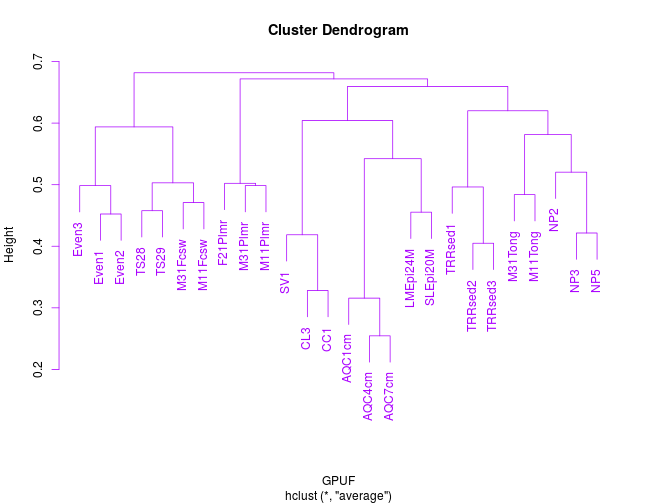An alternative means of summarizing a distance matrix via hierarchical clustering and plotting as an annotated dendrogram. Compare with Figure 4 from the Global Patterns). Some differences in Figure~\ref{fig:GPfig4 from the original article might be explained by Global Patterns in phyloseq being the summed observations from both primer directions (5' and 3'), while in the article they show the results separately. Furthermore, in the article the “mock” community is not included in the dataset, but an extra fecal sample is included.

## Validation

### Multiple Inference Correction

The phyloseq package includes support for significance testing with correction for multiple inference. This is particularly important when testing for significance of the abundance patterns among thousands of microbes (OTUs). This is a common question of phylogenetic sequence data, that is, “what is the subset of microbes that significantly correlate with a scientifically-interesting sample variable”. Although we plan to include support for other types of multiple-inference corrected tests, this “which taxa?” test is the only directly supported test at the moment.

Our initial implementation of this support is via an extension to the mt.maxT and mt.minP functions in the multtest package (Bioconductor repo). This uses permutation-adjusted p-values in a multiple testing procedure that provides strong control of the Family-Wise Error Rate (FWER) among the taxa being tested. The user specifies a sample-variable among the sample_data component, or alternatively provides a sample-wise vector or factor that classifies the samples into groups. Additional optional parameters can be provided that specify the type of test ("test="), the sidedness of the test ("side="), as well as some additional technical/computational parameters.

In the following example we test whether a particular genera correlates with the Enterotype classification of each sample. Note that we have to specify an alternate test, test="f", because the default test (t-test) can only handle up to 2 classes, and there are three enterotype classes.

data(enterotype)
# Filter samples that don't have Enterotype classification.
x <- subset_samples(enterotype, !is.na(Enterotype))